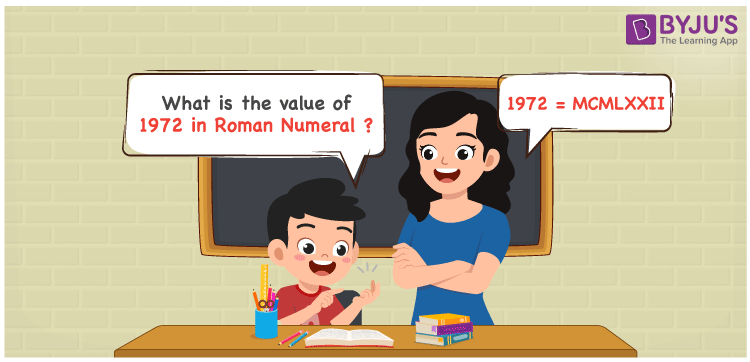# 1972 in Roman Numerals

1972 in Roman Numerals is MCMLXXII. Any Roman numeral uses only the 7 primary symbols which are I, V, X, L, C, D, and M, Where M represents 1000, C represents 100, L represents 50, X represents 10 and I represents 1. MCMLXXII represents the number 1972 which is obtained by the addition and subtraction rules of Roman Numerals. What are Roman numerals? can be referred for more details.

 Number Roman Numeral 1972 MCMLXXII

## How to Write 1972 in Roman Numerals?To convert 1972 in Roman Numerals, we need to represent 1972 as the sum of the fundamental symbols. 1972 can be broken down as

1. The number 1000,
2. Difference of 1000 and 100 to get 900
3. Add 50, to twice of 10 to get 70
4. Add 1 and 1 to get 2
5. Add all the numbers from previous steps.

Let us understand it with Numerical representation.

1972 = 1000 + 900 + 70 + 2

1972 = 1000 + (1000 – 100) + (50 + 10 + 10 ) + ( 1 + 1)

1972 = M + (M – C) + (L + X + X) + (I + I)

1972 = M + (CM) + LXX + II

1972 = MCMLXXII

## Video Lesson on Roman Numerals## Frequently Asked Questions on 1972 in Roman Numerals

Q1

### How to write the number 1972 in Roman Numerals?

1972 in Roman Numerals is written as MCMLXXII.
Q2

### Does MCMLXXII represent Roman Numerals?

Yes. MCMLXXII represents Roman Numerals and it is the number 1972
Q3

### What is the year 1972 in Roman numerals?

The year 1972 in Roman Numerals is MCMLXXII.